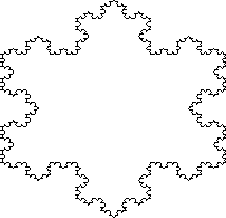Next: Capacity Dimension Up: FRACTAL DIMENSIONS Previous: FRACTAL DIMENSIONS

# Defining Fractals and Fractal Dimensions

Fractals are sets that appear to have complex structure no matter what scale is used to examine them. True fractals are infinite sets and have self-similarity across scales, so that the same quality of structure is seen as one zooms in on them. Figure 2 shows a finite set of one such well-behaved fractal called a Koch curve.If one wants to know the length of the Koch curve, it can be derived from its construction formula. But such computations cannot be done for fractals in nature, such as the outline of a cloud, the outline of a leaf or coastlines. For example, there is no construction process or a formula for the coastline of Great Britain. The only way to get the length of the coastline is to measure it. We can measure the coast on a geographical map by taking rulers set at a certain length. For a scale of 1:1,000,000 meters, the ruler length of 5 cm would be 50 km. Now we can walk this ruler along the coast. This would give a polygonal representation of the coast of Britain as shown in Figure 3. To obtain the length of the coast, we can count the number of steps, multiply the number of steps with 5 cm and convert the result to km. Smaller settings of the rulers would result in more detailed polygons and surprisingly bigger values of the measurements. This can be clearly seen in Table 1 which lists the length measurements of the coast of Britain for different ruler settings (Peitgen, Jurgens & D Saupe,1992). One can also conclude from Table 1 that for ruler settings smaller than 65.40 km the length would have even higher values.Ruler Setting(km) Length(km) 500.00 2600 258.82 3800 130.53 5770 65.40 8640

A similar measurement of length for a circle shows much less variation in the length when the ruler setting is changed. Table 2 shows such measurements for a circle with a diameter of 1000 km.

 Number of Sides Ruler Setting(km) Length(km) 6 500.00 3000 12 258.82 3106 24 130.53 3133 48 65.40 3139 96 32.72 3141 192 16.36 3141

Curves, surfaces, and volumes can be so complex that the ordinary measurements like length, area and volume become meaningless. However, one can measure the degree of complexity by evaluating how fast these measurements increase if we measure with smaller and smaller scales. The fundamental idea is to assume that the measurement and the scale do not vary arbitrarily but are related by a power law which allows us to compute one from the other. The power law can be stated as, where x is the scale used to measure the quantity y and d is a constant. d is a useful quantity in describing fractal dimensions. In the beginning of the twentieth century, determining the meaning of dimensions was one of the major problems. Since then the topic has become more complex because now there are many more notions of dimensions. Some of theses notions are reviewed by Mayer-Kress (1986), Schuster (1988) and Gershenfeld (1988).

Fractal dimensions are always smaller than the number of degrees of freedom (Grassberger & Procaccia 1983) i.e. they are smaller than 2 for two dimensional geometrical objects, smaller than 3 for three dimensional data etc. In simple terms, they can be looked at as indications of how much closer to a geometrical dimension a given set is. All the different dimensions are related. Sometimes they agree with each other, giving the same values, and sometimes they differ from each other depending on the distribution of the set they are being applied to. In this project we used capacity dimension and correlation dimension.Next: Capacity Dimension Up: FRACTAL DIMENSIONS Previous: FRACTAL DIMENSIONS
Sandip Thanki
1999-07-29